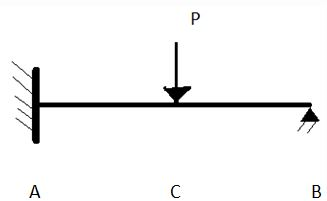Courses

# Test: Force Method of Analysis: General Procedure

## 10 Questions MCQ Test Structural Analysis | Test: Force Method of Analysis: General Procedure

Description
This mock test of Test: Force Method of Analysis: General Procedure for Civil Engineering (CE) helps you for every Civil Engineering (CE) entrance exam. This contains 10 Multiple Choice Questions for Civil Engineering (CE) Test: Force Method of Analysis: General Procedure (mcq) to study with solutions a complete question bank. The solved questions answers in this Test: Force Method of Analysis: General Procedure quiz give you a good mix of easy questions and tough questions. Civil Engineering (CE) students definitely take this Test: Force Method of Analysis: General Procedure exercise for a better result in the exam. You can find other Test: Force Method of Analysis: General Procedure extra questions, long questions & short questions for Civil Engineering (CE) on EduRev as well by searching above.
QUESTION: 1

###In above figure, joint A is fixed and joint B is pinned. C lies in between A and B and a load of P are applied there. Δb = Displacement caused when vertical support at point B is chosen to be neglected. By = redundant force Q.How many unknowns are there in the above figure?

Solution:

Explanation: 4 unknown forces are there, 3 forces and one moment.

QUESTION: 2

###In above figure, joint A is fixed and joint B is pinned. C lies in between A and B and a load of P are applied there. Δb = Displacement caused when vertical support at point B is chosen to be neglected. By = redundant force Q.The above structure is statically indeterminate. State whether the above sentence is true or false.

Solution:

Explanation: 4 unknown forces and 3 reactions are there. So, it is statically indeterminate.

QUESTION: 3

###In above figure, joint A is fixed and joint B is pinned. C lies in between A and B and a load of P are applied there. Δb = Displacement caused when vertical support at point B is chosen to be neglected. By = redundant force Q.  This structure is made redundant by temporarily removing how many support reactions?

Solution:

Explanation: As the degree of indeterminacy is one, one of the support reactions is chosen to be redundant.

QUESTION: 4In above figure, joint A is fixed and joint B is pinned. C lies in between A and B and a load of P are applied there.
Δb = Displacement caused when vertical support at point B is chosen to be neglected.
By = redundant force
Q.  Which of the following forces can’t be chosen to be redundant?

Solution:

Explanation: Load P can’t be chosen as this is the load causing deflection. Rest all support forces can be chosen to be redundant.

QUESTION: 5In above figure, joint A is fixed and joint B is pinned. C lies in between A and B and a load of P are applied there.
Δb = Displacement caused when vertical support at point B is chosen to be neglected.
By = redundant force
Q.  Δ/ bb refers to displacement cause by By.
What does first b in Δ/ bb stands for?

Solution:

Explanation: First b refers to the point where load is specified, second B refers to the point where load is applied.

QUESTION: 6In above figure, joint A is fixed and joint B is pinned. C lies in between A and B and a load of P are applied there.
Δb = Displacement caused when vertical support at point B is chosen to be neglected.
By = redundant force
Q. What is the compatibility equation for the above mentioned condition?

Solution:

Explanation: Both the displacement will be opposite in direction and equal in magnitude.

QUESTION: 7In above figure, joint A is fixed and joint B is pinned. C lies in between A and B and a load of P are applied there.
Δb = Displacement caused when vertical support at point B is chosen to be neglected.
By = redundant force
Q. How much load acts and in which direction at point B for the displacement to be fbb?

Solution:

Explanation: Displacement caused by unit load acting in the direction of By is termed as fbb.

QUESTION: 8In above figure, joint A is fixed and joint B is pinned. C lies in between A and B and a load of P are applied there.
Δb = Displacement caused when vertical support at point B is chosen to be neglected.
By = redundant force
Q. Which one of the following describes fbb?

Solution:

Explanation: Δ/ bb is actual displacement caused by By, and fbb is caused by unit load. So, its fbb times By Δ/ bb/By.

QUESTION: 9In above figure, joint A is fixed and joint B is pinned. C lies in between A and B and a load of P are applied there.
Δb = Displacement caused when vertical support at point B is chosen to be neglected.
By = redundant force
Q. According to maxwell’s theorem:-

Solution:

Explanation: The displacement of a point B on a structure due to a unit load acting at point A is equal to the displacement of point A when the unit load is acting at point B

QUESTION: 10In above figure, joint A is fixed and joint B is pinned. C lies in between A and B and a load of P are applied there.
Δb = Displacement caused when vertical support at point B is chosen to be neglected.
By = redundant force
Q. Maxwell’s theorem doesn’t apply when external moments are placed on the beam.
State whether the above statement is true or false.

Solution: